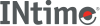﻿ floorINtime SDK Help
floor

Calculates the floor (largest integer that is less than or equal to a value) of a double value.

```#include <math.h>

double floor (double x);
```

#### Parameters

`x`
Value to calculate the floor for.

#### Return Values

The floor result.

#### Requirements

Versions Defined in Include Link to
INtime 3.0 intime/rt/include/math.h math.h clib.lib